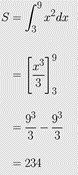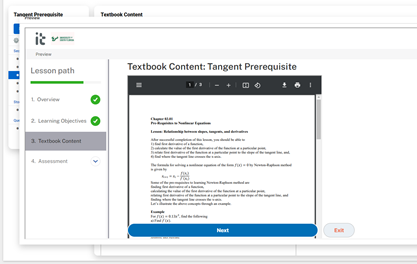## Removing YAML from an RMD file through an R script

So you want to write a book from many RMD files using bookdown.  Here is an R script that strips the YAML lines of an Rmd file because bookdown does not accept files with its own YAML. A new file is created that has no YAML.  The program is written just for one Rmd file, and I will be writing an extension of it to do this for a whole directory including subdirectories.  I will soon write a script that will move the YAML absent RMD files and associated sub-directories to a new directory of its own.

# A program to remove YAML from an RMD file.
# It can be modified to do this for all
# files in a directory and/or its subdirectories
# Stay tuned for the update.

#Set the working directory
setwd("C:/Users/yoda/Rmd")

# filename of the rmd file whose YAML you want to take out
fileName <- "Chapter01.03RoundoffErrors/0103RoundOffErrorsYaml.rmd"

# Open the file to read
Input_File <- file(fileName,open="r")

# icapture is a vector which will check the two lines
# that have --- in them.
icapture <- vector(,10)

# Just printing the lines in the rmd file, not needed.
#for (i in 1:length(linn)){
# print(linn[i])
#}

#The name of the file which will store YAML free RMD file.
YAML_Remove_File <- file("Chapter01.03RoundoffErrors/0103RoundOffErrorsYamlRemove.rmd",open="w")

j <- 0
#Capturing the two line numbers where --- exists
for (i in 1:length(linn)){
if(strtrim(linn[i],3) == "---"){
j <- j+1
icapture[j] <- i
}

# Write to the output file only if it has already captured two ---
if ((j==2) & (i!=icapture)){
writeLines(linn[i],con2)
}
}

#close the input and output files
close(Input_File)
close(YAML_Remove_File)

____________________________

This post is brought to you by

• Holistic Numerical Methods Open Course Ware:
• the textbooks on
• the Massive Open Online Course (MOOCs) available at

## Useful hints for a newbie on Rmarkdown

An R Markdown newbie walks on eggshells so as to not look naïve.  But the community is so nice to them, and since the help is all in the open, it reaches many more.  So here are a few items I learned beyond the usual R Markdown and the ubiquitous example about plotting something about cars.

##### Not Autonumbering

Don’t want to autonumber. If you write (1) First, it will automatically start numbering it. Convenient, but I do not like it sometimes. So how does one stop items from autonumbering. Just put a period sign after (1), that is, (1).  Here is an example.  This will keep the numbering the same as what is inputted.

(1).first
(2).second
(2).second
(3).numbering went away
##### Not wanting to center a block equation

Do you not want a block equation (an equation on its own separate line as opposed to being part of the text which is called an inline equation) to not be in the center, but wanted it tabbed instead.  It is easy to center equations by writing them within two double dollars symbols, that is, .  But I do not like centering.  I like my block equations tabbed.

The equation
$latex a_{11} x_1+a_{12} x_2+\cdots+a_{1n}x_n=b_1$
gets centered by entering as

$$a_{11} x_1+a_{12} x_2+\cdots+a_{1n}x_n=b_1$$

The following, however, put tabs in the equation. Each &emsp puts 4 spaces.  Note also the two dollar symbols, that is, , bounding the equation

&emsp;&emsp;$a_{11} x_1+a_{12} x_2+\cdots+a_{1n}x_n=b_1$
##### Defining often used equations

If you are using certain equation parts, again and again, you can define them.  See  here we are defining $latex \overline{X}$ and $latex \sum_{i=1}^{n}$

{=tex}
\def\Xbar{\overline{X}}
\def\sumn{\sum_{i=1}^{n}}

##### Aligning equations to a character

Many times, you may have equations that are aligned by a character, say an equal to sign.  But if the equations get centered, the equal to sign may not get centered.  This is simply done by adding a & before the aligned character in all lines.  For example, if you want to show the following,you would enter it as the following.  Note where the & is.

\begin{align} \ S &= \int_{3}^{9}{x^2 dx}\\ &= \left[\frac{x^3}{3}\right]_3^9\\ &= \frac{9^3}{3}-\frac{9^3}{3}\\ &= 234 \end{align}

____________________________

References: An Example R Markdown http://statpower.net/Content/311/R%20Stuff/SampleMarkdown.html

This post is brought to you by

• Holistic Numerical Methods Open Course Ware:
• the textbooks on
• the Massive Open Online Course (MOOCs) available at

## Converting a Word docx file to a draft R Markdown file

Many of have been using MS Word as a word processor for decades now.  What is then an R Markdown document?  An R Markdown document is written in markdown (fancy way of saying that it is all in plain text) and embedded in it can be chunks of R code.  Once written, you can render the file into many formats including HTML, MS Word and PDF.  So, why would someone like me choose to convert a MS Word file to a R Markdown file.  Isn’t MS Word enough to meet my needs?  I have two good reasons to convert my MS word files of my open-resource Numerical Methods course to R Markdown files.

1. On conversion from the MS Equation editor 3.0 to the currently available MS equation editor for .docx files, the equations from my old .doc documents  were getting displayed in a compact inline form.  Using the display option of an equation would have supposedly helped, but some equations refused to get properly justified, tabbing was becoming a guessing game, and using a created Word style was not helping.  Sometimes, equations would not show with letters italicized, and italicizing a single equation would change the whole document to italics font.  Ctrl+Z would help in un-italicizing the document but that was not foolproof either as it would sometimes mess up the tabs.
2. The second reason was that I was embedding PDF files in a frame in an online adaptive platform lesson and even with a 12-point size in the original document, the font of the PDF files would show up too small (see Figure 1).  Yes, one can use a bigger font size in the Word file but this may not be suitable for use in, say, a printed textbook.  Maintaining different versions with different font sizes is not a recommended practice in today’s world.  A user could alternatively use the magnification option of the PDF file menu, but that creates horizontal scrolls as well in the frame.  Also, a user could download the PDF file to be opened in an acrobat reader but that is an  inconvenience imposed on them.  One could also simply embed an .htm version of the word file but such file content was getting rendered all over the place as my documents included equations, both in inline and display modes, sketches made with Word, tables imported from excel, and plots obtained from a MATLAB output, etc.Figure 1: Embedded PDF file shows up with a small font

So the answer was simply to take Rmarkdown for a spin.  Since our documents are not simply text, it is not a cut-and-paste job with some light editing.  We turned to pandoc for this.  What pandoc is can be summarised by their slogan – “If you need to convert files from one markup format into another, pandoc is your swiss-army knife”.  Pandoc is a free software and is released under the GPL.  The full manual for pandoc is also available.

Here are the steps for how to do the conversion on a Windows 10 machine.  One has to do the conversion though at the command prompt level as I did not see an online converter that does the conversion beyond text and styles, that is, they do not convert equations, images, etc.

2. Install pandoc as an administrator.
3. Check if Pandoc is installed.  Go to the search box in your taskbar and enter “cmd” without the quotes.  Run as administrator.  You will get the cmd prompt.  At the prompt enter “pandoc –version” without the quotes.
4. Go to the command prompt by entering “cmd” without the quotes in the search box in your taskbar.
5. Go to the directory where the .docx file is stored.  You can do this by use cd and cd.. commands.   See here for a short guide.
6. Once in the directory, do the following.  Let’s suppose the name of the file is “Chapter01NumericalMethods.docx”.   Type the following at the command prompt.
• pandoc --extract-media ./Chapter01NumericalMethodsMedia "Chapter01NumericalMethods.docx" -t markdown -o "Chapter01NumericalMethodsOut.md"
• The above format extracts the media files as well and puts them in a media directory ./Chapter01NumericalMethodsMedia.  Some files may be of the .wmf format.  These can be opened in MS Paint and saved in an acceptable format such as .png.
• I always use quotes for file names to avoid errors one gets with spaces in filenames, etc.
• It is good practice to use a different name for the output markdown file as one may later be converting the markdown files to different formats including pdf, HTML, word, etc.  Note the output markdown file is with an .md suffix as pandoc does not have the output .rmd option.
7. Rename the .md file as .rmd file
8. Open the .rmd file in Rstudio to edit.

The above is a recipe for just one file.  I do gather if one has many .docx files, one could write a script to do this in a batch mode.

We will discuss some tricks to light edit the .rmd file in the next blog.  Stay tuned on the journey of this Rmarkdown newbie.  If you know a better way to do this, please let me know – autarkaw at yahoo.com.

____________________________

This post is brought to you by

• Holistic Numerical Methods Open Course Ware:
• the textbooks on
• the Massive Open Online Course (MOOCs) available at